News

December 10, 2020 by Dr. Steve Arar

### Analog Device’s New CTSD ADC

Analog Devices has announced a new 24-bit, 4-channel, precision ADC, AD7134, that is said to simplify signal chain design by eliminating the need for an external anti-alias filter. The new device, which supports a scalable output data rate from 10 SPS to 1.5 MSPS, employs a continuous-time sigma-delta (CTSD) architecture to enable an inherent alias rejection of up to 102.5 dB.

With no anti-aliasing filter in the signal chain, it is possible to reduce the signal chain latency as well as the solution size. Analog Devices claims that the new product can save board space by 70% compared to a traditional design.

Another advantage of eliminating the external anti-aliasing filter is a higher phase matching in multi-channel systems. Besides, with the new architecture, we can avoid performance worries such as offset, gain error, and noise that come along with an external filter. The functional block diagram of the AD7134 is shown below.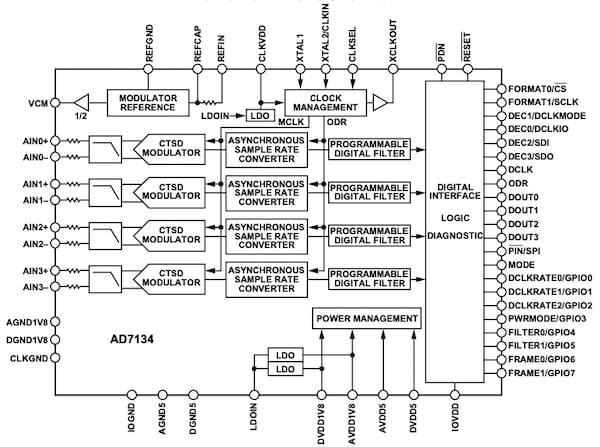##### Functional block diagram of the AD7134. Image courtesy of Analog Devices

It may be useful to now review some basic concepts of sigma-delta ADCs. Then, we'll briefly compare two possible implementations: continuous-time sigma-delta (CTSD) and discrete-time sigma-delta (DTSD) architectures.

### Block Diagram of a Sigma-Delta ADC

As depicted below, a sigma-delta ADC consists of two primary blocks: a sigma-delta modulator and a digital filter.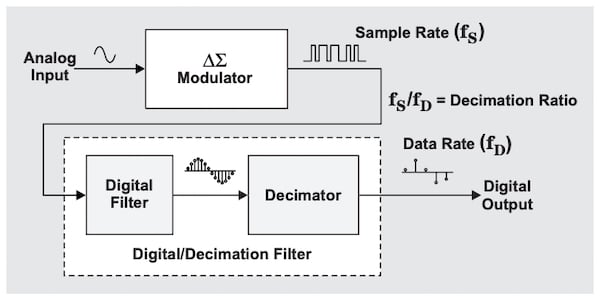##### Block diagram of a sigma-delta ADC.Image courtesy of Texas Instruments

With a 1-bit sampling system, the sigma-delta modulator converts the analog input to a high-speed stream of single-bit values.

How can this stream of single-bit values represent the analog input value? The sigma-delta modulator oversamples the input and the single-bit values are output at a much much higher rate than that required by the Nyquist sampling criterion.

Many of the consecutive bit values (for example, 64 of them) should be averaged to get a single multi-bit value that represents the analog input. In other words, the ratio of the number of ones to zeros in the stream of single-bit values represents the input analog voltage.

This averaging process takes place in a digital filter that is explicitly shown in the above block diagram. Then, a decimator filter (which I discuss in more detail in my article on multirate DSP in A/D conversion) discards some of the samples to reduce the sample rate to a lower value without losing the information we’re interested in.

The sampling process adds a noise component known as quantization noise to the input signal. The total power of the quantization noise is determined by the LSB size of the quantizer. In the frequency domain, this total noise power will spread over the frequency range from DC to half the sampling frequency, $$\frac{f_s}{2}$$.

Therefore, for a given LSB size, we can increase the sampling frequency relative to the input signal bandwidth to reduce the noise power in the frequency band of interest. This is one of the basic techniques that allow a sigma-delta ADC to have a high signal-to-noise ratio (SNR).

Another fundamental technique that enables a high SNR is noise shaping. To combat the quantization noise, a sigma-delta modulator shapes the noise power profile so that most of the noise power moves to higher frequencies outside of the bandwidth of interest.

The figure below shows how a sigma-delta ADC uses oversampling and noise shaping to reduce the quantization noise in the frequency band of interest.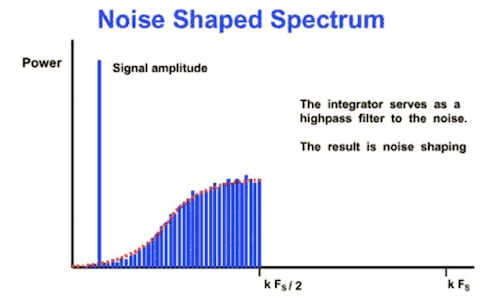### Two Different Implementations

We can use both discrete-time and continuous-time blocks to implement a sigma-delta modulator. The following figure conceptually shows these two different implementations.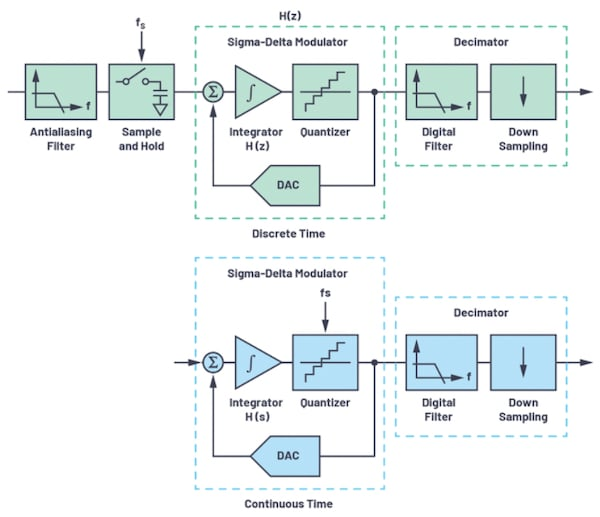##### Schematics depicting discrete-time and continuous-time modular blocks. Image courtesy of Analog Devices

With a discrete-time modulator, a sample-and-hold block should be placed in front of the modulator and the modulator building blocks, such as the loop filter H(z) and DAC, are switched-capacitor based circuits. However, with a continuous-time implementation, the loop filter H(s) and DAC are continuous-time circuits and sampling occurs within the quantizer after the loop filter.

### The Advantages of a Continuous-Time Modulator

A CTSD offers several advantages over a DTSD.

First, with a continuous-time structure, the sampler is placed after the loop filter. As a result, the loop filter can now act as an anti-aliasing filter in addition to its original purpose, which was shaping the power profile of the quantization noise. Therefore, with a CTSD, we might be able to totally eliminate the external anti-aliasing filter or at least relax its requirements significantly. Eliminating the external filter can dramatically save board area and reduce power consumption.

Another important advantage of a CTSD over a DTSD is that the input impedance of a continuous-time solution is resistive. This contrasts a DTSD where the impedance “seen” by the anti-aliasing filter is capacitive.

The capacitive input of a DTSD can cause issues because when a new sample is being taken, the charge previously stored in the sampling capacitor can temporarily distort the input. These switching kickbacks can occur both at the analog input and the reference input of the ADC. Hence, high-bandwidth driving amplifiers are commonly required to supply the charging/discharging transient currents of the inputs of a DTSD.

Since the analog and reference inputs of a CTSD are resistive, they are much quieter compared to capacitive inputs of a DTSD. With no switching kickback, we can remove the driving amplifier, which further simplifies the design and reduces power consumption. As illustrated below, the signal chain of CTSD is much simpler than that of a DTSD.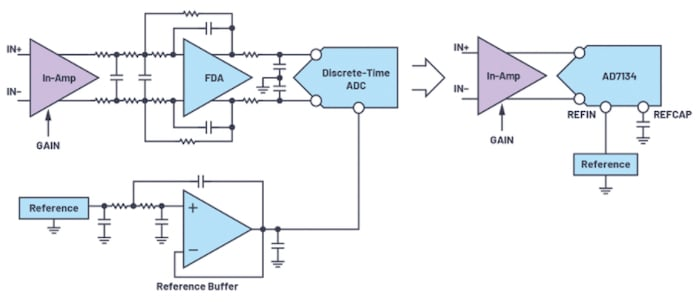##### Comparison of a discrete-time-based and a continuous-time-based signal chain. Image courtesy of Analog Devices

However, the dynamics of the continuous-time integrators employed in a CTSD architecture is determined by the product of two different parameters—for example, the RC product in an active-RC filter and the $$\frac{G_m}{C}$$ value in the case of a Gm-C filter. Consequently, the sampling frequency of a CTSD will be usually fixed.